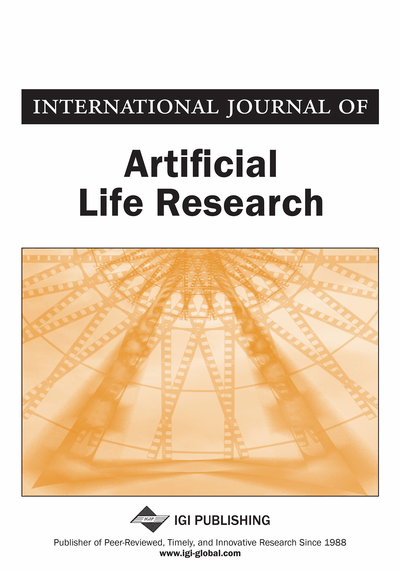# Preinvexity and Semi-Continuity of Fuzzy Mappings

Yu-Ru Syau (National Formosa University, Taiwan) and E. Stanley Lee (Kansas State University, USA)
DOI: 10.4018/jalr.2010070106
Available
\$37.50
No Current Special Offers

## Abstract

In this paper, the relationships between semicontinuity and preinvexity of fuzzy mappings are investigated. The concepts of intermediate-point preinvex fuzzy mappings and weakly preinvex fuzzy mappings are first introduced, and the authors establish a characterization for a weakly preinvex fuzzy mapping in terms of its epigraph. In this context, a characterization of a preinvex fuzzy mapping in terms of its relationship with intermediate-point preinvexity and weakly preinvexity, respectively, is obtained.
Article Preview
Top

## 1. Introduction

Convexity and semicontinuity of fuzzy mappings play a central role in the theories of fuzzy optimization and fuzzy nonlinear programming. Various investigators (Furukawa, 1998; Nanda & Kar, 1992; Syau, 1999; Wang & Wu, 2003) studied and developed criteria for convex fuzzy mappings. The concept of upper and lower semicontinuity of fuzzy mappings based on the Hausdorff separation was introduced by Diamond and Kloeden (1994). Recently, Bao and Wu (2006) introduced new concepts of upper and lower semicontinuous fuzzy mappings through the “fuzz-max” order on fuzzy numbers in ways that parallel closely to the corresponding definitions for upper and lower semicontinuous real-valued functions. They also obtained criteria for convex fuzzy mappings under upper and lower semicontinuity conditions. In an earlier paper (Syau, Sugianto, & Lee, 2008), we redefined the upper and lower semicontinuity of fuzzy mappings of Bao and Wu (2006) by using the concept of parameterized triples of fuzzy numbers.

Noor (1994) extended the concept of preinvexity to fuzzy mappings. It was proved in Noor (1994) and our earlier paper (Syau, 1999) that preinvex fuzzy mappings are more general than convex fuzzy mappings, and that preinvexity can be substituted for convexity in many results in mathematical fuzzy programming involving convex fuzzy mappings.

Motivated by earlier research works, and by the importance of preinvexity and semicontinuity of fuzzy mappings, the relationships between semicontinuity and preinvexity of fuzzy mappings are investigated in this study. We introduce the concepts of intermediate-point preinvex fuzzy mappings and weakly preinvex fuzzy mappings. We also give a characterization for a weakly preinvex fuzzy mapping in terms of its epigraph. A characterization of a preinvex fuzzy mapping in terms of its relationship with intermediate-point preinvexity and weakly preinvexity, respectively, is obtained.

Top

## 2. Preliminaries

In this section, for convenience, several definitions and results without proof from (Bao & Wu, 2006; Mohan & Neogy, 1995; Noor, 1994; Syau et al., 2008; Weir & Jeyakumar, 1988; Weir & Mond, 1988; Yang, 2001) are summarized below.

wherebeing the Euclidean norm on.

First, we recall the definitions of upper and lower semicontinuous real-valued functions.

## Complete Article List

Search this Journal:
Reset
Open Access Articles: Forthcoming
Volume 8: 2 Issues (2018)
Volume 7: 2 Issues (2017)
Volume 6: 2 Issues (2016)
Volume 5: 1 Issue (2015)
Volume 4: 1 Issue (2014)
Volume 3: 4 Issues (2012)
Volume 2: 4 Issues (2011)
Volume 1: 4 Issues (2010)
View Complete Journal Contents Listing